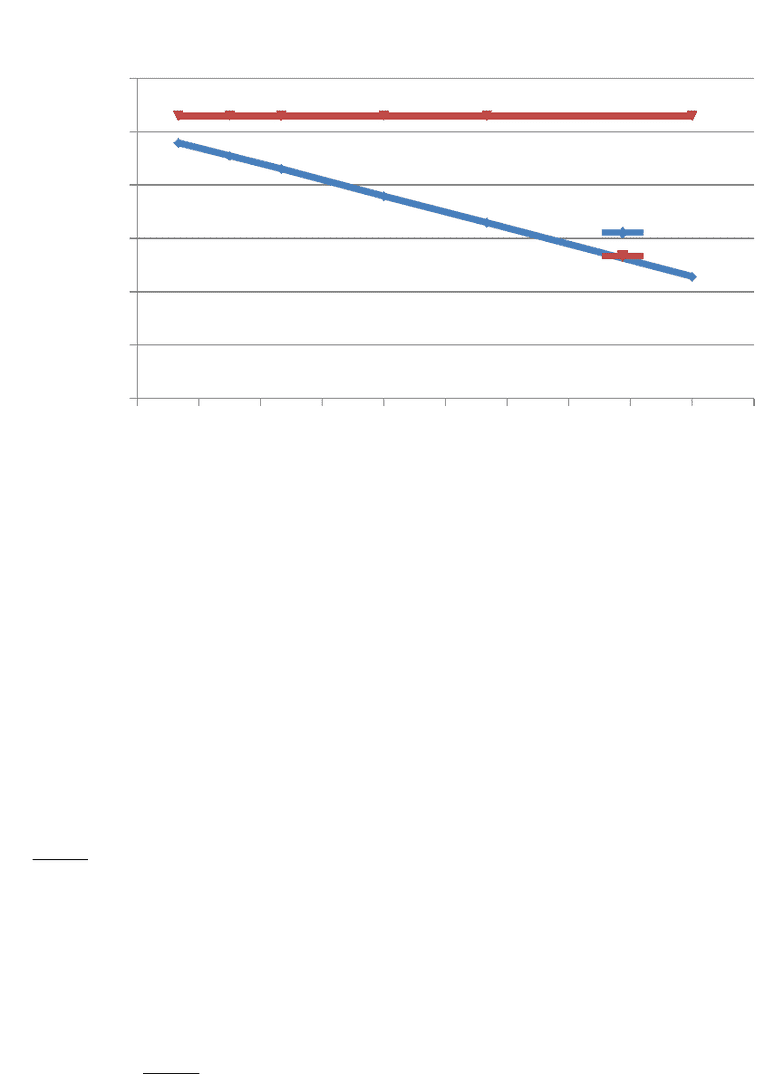# CHM110H5 Final: Assignment 2

29 views3 pages
School
Department
Course
ProfessorAssignment #: 2
a)
0.1 0.2 0.3 0.4 0.5 0.6 0.7 0.8 0.9 1 1.1
22.15
22.2
22.25
22.3
22.35
22.4
22.45
PV as a function of P for 1.0 mol CO2 at
V CO2 (L)
V Ideal Gas
(L)
Pressure (atm)
Pressure . Volume (L atm)
Figure 1: V CO2 (diamond); X is pressure with the unit atm, and Y is Pressure*Volume
with the unit Latm. There is a decreasing trend in this function. As the value of P
increases, the value of PV decreases. Thus the equation, PV=22.415-0.158P. V Ideal
Gas (Square); X is pressure with unit atm, and Y is Pressure*Volume with the unit
Latm. The function is constant, as the values of P increase the value of PV remains
22.4125. Thus the equation, PV=22.415. The graph corresponds to the equation:
PV=RT+BP; where PV is the value of Y, RT is the value of the Y-intercept, B is the
slope and P is the x value. The
b) RT is the y-intercept of the above equation. When pressure is equal to 0 atm, the RT
value (y-intercept) is equal to the value of how much PV is found in the gas CO2. This
can be confirmed by determining the units of RT:
RT=
(
L atm
mol K
)
(
K
)
The K values cancel each other out, and since 1 mol is being considered the units of RT
are
RT=L atm
.
Determining the value of RT:
The ideal gas law: PV=nRT1, considering 1 mol:
Therefore, the equation becomes PV=RT. R is a constant, 0.08205 and T is given,
273K 2
PV=(0.08205
(
L atm
mol K
)
(273 K)
Unlock document

This preview shows page 1 of the document.
Unlock all 3 pages and 3 million more documents.